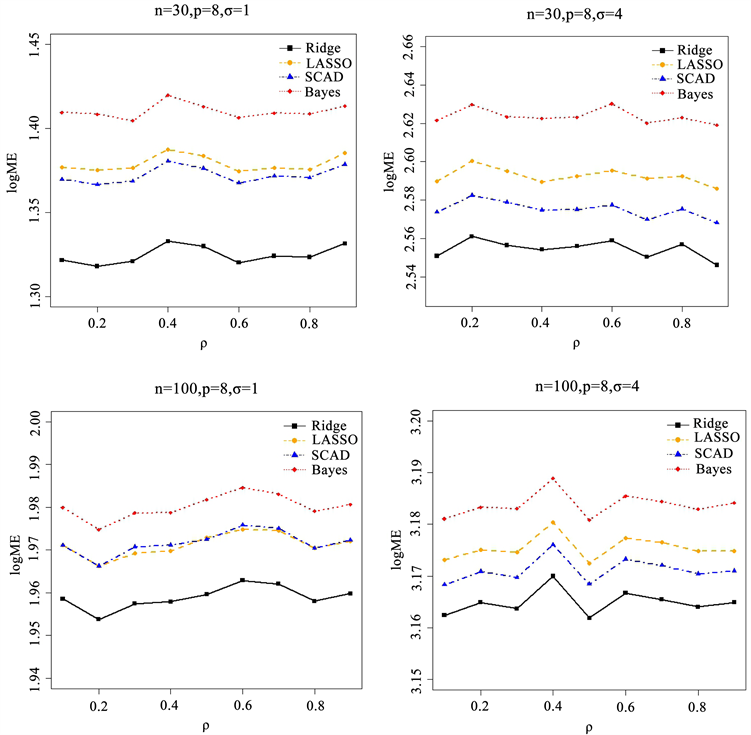# 稀疏模型下的模型选择方法比较及应用Application and Comparison of Model Selection Methods under Sparse Models

DOI: 10.12677/SA.2018.75062, PDF, HTML, XML, 下载: 527  浏览: 2,187

Abstract: In this paper, we study the model selection in multiple linear regressions. Model selection is a hot topic in statistical research. With the advent of the era of large data, the dimension of data is getting higher and higher. There is a greater demand for model selection in the fields of economics and finance, biostatistics and image processing. At the same time, sparse model plays an increasingly important role in machine learning, which can avoid over-fitting. This paper mainly studies the model selection based on multiple linear regression model, and summarizes the ridge regression, LASSO and SCAD methods and Bayesian model selection methods. Later, through data simulation and case analysis, we focus on the sparse model under the premise of the four model selection methods that are analyzed and compared. Through research and analysis, it can be found that the results of ridge regression are better when the model is approximately sparse, SCAD method can better remove the unimportant variables, and the results are very little different from ridge regression. Therefore, this good property can be fully utilized in practical application.

1. 引言

${Y}_{i}={x}_{i}^{T}\beta +{\epsilon }_{i}$ ，或者等价于 $Y=X\beta +\epsilon$

2. 惩罚因子模型选择

$Y=X\beta +\epsilon$ (1)

${\stackrel{^}{\beta }}_{OLS}=\mathrm{arg}\mathrm{min}{‖Y-X\beta ‖}^{2}={\left({X}^{\prime }X\right)}^{-1}{X}^{\prime }Y$ (2)

${‖Y-X\beta ‖}^{2}+{P}_{\lambda }\left(|\beta |\right)$ (3)

2.1. 岭回归

${\stackrel{^}{\beta }}_{ridge}={\left({X}^{\prime }X+\lambda {I}_{p}\right)}^{-1}{X}^{\prime }Y$ (4)

${\stackrel{^}{\beta }}_{ridge}=\mathrm{arg}\mathrm{min}\left\{{‖Y-X\beta ‖}^{2}+\lambda \underset{j=1}{\overset{p}{\sum }}{\beta }_{j}^{2}\right\}$ (5)

$GCV\left(\lambda \right)=\frac{{‖\left({I}_{n}-A\left(\lambda \right)\right)Y‖}^{2}}{{\left(Trace\left({I}_{n}-A\left(\lambda \right)\right)\right)}^{2}}$ (6)

2.2. LASSO方法

LASSO方法是Tibshirani  在The nonnegative garrote (NG)  方法的基础上发展而来的，也是岭回归的一特殊形式。对于NG方法，其参数估计为

${\stackrel{^}{\beta }}_{NG}=\left({u}_{1}{\stackrel{^}{\beta }}_{1},\cdots ,{u}_{p}{\stackrel{^}{\beta }}_{p}\right)$ (7)

$\underset{u\ge 0}{\mathrm{min}}\left\{{‖Y-X\stackrel{^}{B}u‖}^{2}+2\lambda \underset{j=1}{\overset{p}{\sum }}{u}_{j}\right\}$ (8)

${\stackrel{^}{\beta }}_{LASSO}=\mathrm{arg}\mathrm{min}\left\{{‖Y-X\beta ‖}^{2}+\lambda \underset{j=1}{\overset{p}{\sum }}|{\beta }_{j}|\right\}$ (9)

${\stackrel{^}{\beta }}_{SCAD}=\mathrm{arg}\mathrm{min}\left\{{‖Y-X\beta ‖}^{2}+\underset{i=1}{\overset{p}{\sum }}{P}_{\lambda ,\gamma }\left(|{\beta }_{i}|\right)\right\}$ (10)

${{P}^{\prime }}_{\lambda ,\gamma }\left(\theta \right)=\lambda \left\{I\left(\theta \le \lambda \right)+\frac{\left(\gamma \lambda -\theta \right)}{\left(\gamma -1\right)}+I\left(\theta >\lambda \right)\right\}$ (11)

3. 贝叶斯模型选择

$P\left({M}_{k}|y\right)=\frac{P\left(y|{M}_{k}\right)P\left({M}_{k}\right)}{\underset{l=1}{\overset{k}{\sum }}P\left(y|{M}_{l}\right)P\left({M}_{l}\right)}$ (12)

$\stackrel{^}{M}=\underset{{M}_{k}}{\mathrm{arg}\mathrm{max}}P\left({M}_{k}|y\right)$ (13)

4. 数据模拟

$Y=X\beta +\epsilon ,\epsilon ~N\left(0,{\sigma }^{2}\right)$ (14)Figure 1. Variation of different correlation coefficients under sparse models

$Y=X\beta +\epsilon ,\epsilon ~N\left(0,{\sigma }^{2}\right)$

5. 实例分析

$Y=\underset{i=1}{\overset{8}{\sum }}{\beta }_{i}{X}_{i}$Figure 2. Coefficient variations in almost sparse models under different conditionsTable 1. Correlation coefficient matrix of prediction factorsTable 2. Analysis of intima-media thickness data

6. 总结

  Hoerl, A.E. and Kennard, R.W. (1970) Ridge Regression: Biased Estimation for Nonorthogonal Problems. Technometrics, 12, 55-67. https://doi.org/10.1080/00401706.1970.10488634  Tibshirani, R. (1996) Regression Shrinkage and Selection via the Lasso. Journal of the Royal Statistical Society, Series B, 58, 267-288.  Fan, J. and Li, R. (2001) Variable Selection via Nonconcave Penalized Likelihood and Its Oracle Properties. Journal of the American Statistical Association, 96, 1348-1360. https://doi.org/10.1198/016214501753382273  Luo, S. and Chen, Z. (2013) Extended BIC for Linear Regression Models with Diverging Number of Relevant Features and High or Ultra-High Feature Spaces. Journal of Statistical Planning and Inference, 143, 494-504. https://doi.org/10.1016/j.jspi.2012.08.015  Cho, H. and Fryzlewicz, P. (2011) High Dimensional Variable Selection Viatilting. Journal of the Royal Statistical Society, Series B, 74, 593-622. https://doi.org/10.1111/j.1467-9868.2011.01023.x  Fan, J. and Lv, J. (2008) Sure Independence Screening for Ultrahigh Dimensional Feature Space. Journal of the Royal Statistical Society, Series B, 70, 849-911. https://doi.org/10.1111/j.1467-9868.2008.00674.x  Fan, J. and Lv, J. (2010) A Selective Overview of Variable Selection in high Dimensional Feature Space. Statistica Sinica, 20, 101-148.  Zhang, K., Yin, F. and Xiong, S. (2014) Comparisons of Penalized Least Squares Methods by Simulations. arXiv:1405.1796v1 [stat.CO]  白玥, 田茂再. 几种高维变量选择方法的比较及应用[J]. 统计与决策, 2017(22), 11-16.  李佳蓓, 朱永忠, 王明刚. 贝叶斯变量选择及模型平均的研究[J]. 统计与信息论坛, 2015, 30(8), 20-24.  Breiman, L. (1995) Better Subset Regression Using the Nonnegative Garrote. Technometrics, 37, 373-384. https://doi.org/10.1080/00401706.1995.10484371  Zou, H. (2006) The Adaptive Lasso and Its Oracle Properties. Journal of the American Statistical Association, 101, 1418-1429. https://doi.org/10.1198/016214506000000735  麦考斯, 德鲁伊特, 利凯. R软件教程与统计分析: 入门到精通[M]. 潘东东, 等, 译. 北京: 高等教育出版社, 2015.